# Lorentz factor facts for kids

Kids Encyclopedia Facts

The Lorentz Factor is the name of the factor by which time, length, and "relativistic mass" change for an object while that object is moving and is often written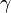$\gamma$ (gamma). This number is determined by the object's speed in the following way: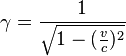$\gamma = \frac{1}{\sqrt{1 - (\frac{v}{c})^2}}$

Where v is the speed of the object and c is the speed of light (expressed in the same units as your speed). The quantity (v/c) is often labeled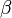$\beta$ (beta) and so the above equation can be rewritten: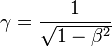$\gamma = \frac{1}{\sqrt{1 - \beta^2}}$

## Classical Relativity

Classical Relativity is the idea that if you throw a ball at 50 mph while running at 5 mph, the ball will now travel 55 mph. Of course, the ball still moves away from you at 50 mph, so if one were to ask you, you saw the ball traveling 50 mph. Meanwhile, your friend Bob saw that you happened to be running at 5 mph. He would say that the ball was traveling 55 mph. Both of you are right, you just happened to be moving with the ball. The speed of light, c, is 670,616,629 mph. So if you are in a car traveling half the speed of light (0.5c) and you turn on your headlights, the light moves away from you at 1 c... or is it 1.5 c? It ends up that c is c no matter what. The next section explains why it's not c - 0.5c.

## Time Dilation

When a clock is in motion, it ticks slower by a (usually small) factor of$\gamma$. The famous twin paradox says that if there were two twins and twin A stayed on earth while twin B traveled near c for a few years, when twin B got back to earth, he would be many years younger than twin A (because he experienced less time). For example, if twin B left when he was 20 and traveled at .9c for 10 years, then we he got back to earth, twin B would be 30 (20 years + 10 years) and twin A would be almost 43: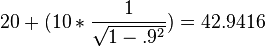$20 + (10*\frac{1}{\sqrt{1-.9^2}}) = 42.9416$

Twin B would not notice that time had slowed at all. To him, if he looked out a window, it would seem like the universe was moving past him, and therefore slower (remember, to him, he's at rest). So time is relative.

## Length Contraction

Another way that the universe changes to make the speed of light not change is that it makes things shorter when they travel. During twin B's journey (see: Time Dilation), he would notice something strange about the universe. He would notice that it got shorter (contracted in the direction of his motion). And the factor by which things get shorter is$\gamma$.

## Relativistic Mass

Something called relativistic mass also increases. It does not actually make things heavier, it just makes them harder to push. So by the time you reach 0.9999c, you need a very big force to make you go faster. This makes it impossible for anything to reach the speed of light.

Still, if you travel a bit slower, say 90% of the speed of light, your mass only grows by 2.3 times. So, while it may be impossible to reach the speed of light, it may still be possible to get close to it—that is, if you have enough fuel.

## Real Consequences

The most significant consequence of these "relativistic effects" for humans is time dilation. If we were to build a space ship capable of traveling very close to the speed of light, the passengers of the ship could travel millions of light years in years or even months, measured with a clock on board the space ship. Because time is relative though, a clock on the observer would see the trip take millions of years.

But, if you travel a bit slower, say 90% of the speed of light, you would take 10% longer to get to your destination, but if a year passed for you, to an outside observer only three years would pass.

The real big effects only take place after you achieve 99% of the speed of light. To get a 1 to 10 relativistic effect (1 year for you passing and 10 years for outside observers) you would need to go about 99% of the speed of light.

And if you wanted a thousand years to pass for an outside observer, while only one would pass for you on the space ship, you would need to be going at 99.9999% the speed of light. And of course your mass would increase a thousand times as well requiring you to carry a whole lot more fuel.Lorentz factor Facts for Kids. Kiddle Encyclopedia.